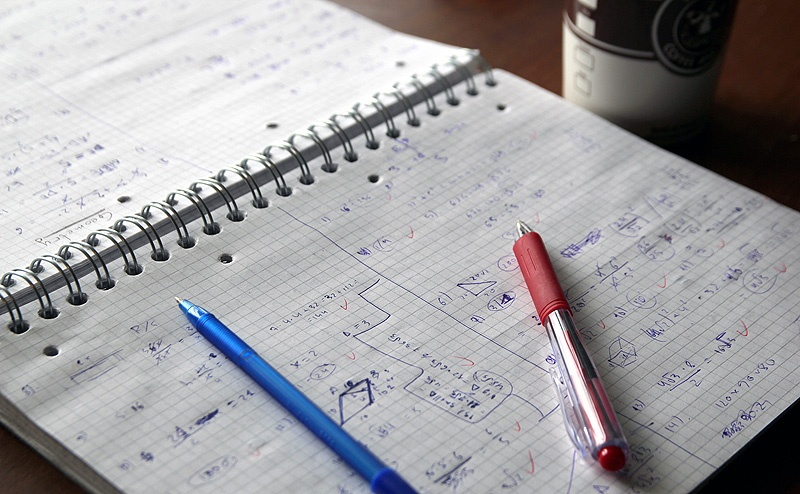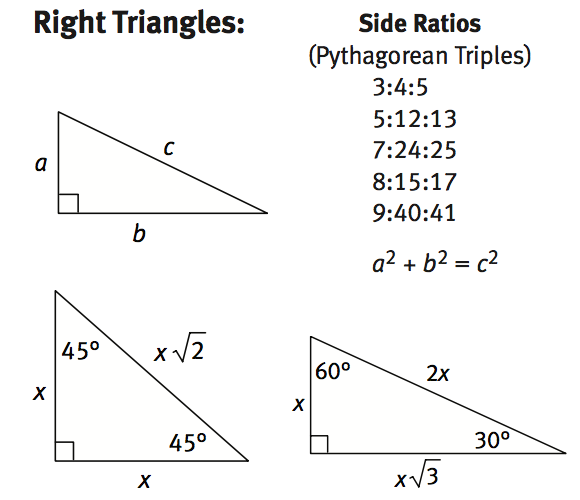# GMAT & MBA Admissions BlogIn our GMAT tutoring sessions, we constantly remind students that the GMAT is not a math test. Although there are some rules you need to know, doing well requires that you think in a structured yet flexible way and approach each question as a problem that needs to be solved strategically and creatively. Rote memorization of facts and formulas is not the answer. Building critical thinking skills is the path to a 700+ GMAT score.

All that said, you will need to memorize some mathematics information before test day. This primer introduces some of those important facts.

### Set the right mental context for what you’ll need to memorize.

It’s important to remember that you will not have a calculator on the GMAT; it will be necessary to memorize a few mathematical equations and rules. Many people feel most overwhelmed with the Quantitative material on the GMAT, but you won’t encounter anything beyond topics you learned in high school. All it takes to master this section is enough dedication to recall and then retain information that you’ve most likely already learned in the past, combined with enough practice in applying these concepts to seemingly very tricky problems using lots of critical thinking, problem solving, and process of elimination.

There is a fair amount of geometry on the GMAT.  You should be prepared to remember facts about various shapes. Draw the shapes when you can – are easier for your brain to process and remember.

Take triangles as an example. You have to remember many equations and rules for different types of triangles (Pythagorean theorem, interior and exterior angles, area, etc.). It can be helpful to list these and draw them as well. Actually draw a right triangle, label the sides (a, b, c) and fill in the fixed angle amounts.Go through the same process for angles, quadrilaterals, circles, angles, and uniform solids.

### Recall your high school algebra equations.

You’ll need to know various math rules and formulas to perform well on the GMAT. Again, these should look familiar from the math classes you took in high school.

For example, how many of the bullets below are familiar to you?

• Order of operations
• FOIL (first, outside, inside, last) when multiple two binomials (i.e., (a +b ) (c + d)
• exponent rules
• Slope of a line, or y = mx + b

MBA Crystal Ball has a great article covering most of the quant section.

### Here are some other helpful GMAT quant issues to remember.

#### Memorize the answer order for data sufficiency questions.

The answers are the same for every question, so the GMAT doesn’t write them out for you. You’ll save lots of time if you’re able to memorize and quickly recall what A, B, C, D, and E represent:

• (A) statement 1 alone is sufficient, but statement 2 alone is not sufficient to answer the question
• (B) statement 2 alone is sufficient, but statement 1 alone is not sufficient to answer the question
• (C) both statements taken together are sufficient to answer the question, but neither statement alone is sufficient
• (D) each statement alone is sufficient
• (E) statements 1 and 2 together are not sufficient, and additional data is needed to answer the question

In situations where all answer choices are numerical, it can be helpful to backsolve (plugging the answers into the question). Start with C, usually the median number, and use it in the equation.  This is an easy way to eliminate answers quickly.

QS Top MBA wrote a nice article summarizing most of what you need to understand to master GMAT data sufficiency questions.

#### Use mental math as often as you can.

If a question uses decimals, (3.2, 4.8, round up to whole numbers to make quick calculations in your head). If you are asked to multiply something by 20% or 30% or 40%, first multiply it by 10% (something most of us can do quickly), and then multiply the result by 2 or 3 or 4. Mental math is all about breaking something that seems complicated on its own into easier steps in your head.

Time management is critical on the GMAT. Taking even an extra 30 seconds to manually work out a problem that can be completed using mental math wastes precious time. It also increases the possibility that you might make a calculation error.

Mental math also has a way of improving your more general math skills. As explained in this by Shelley Gray, a math teacher, it forces you to understand instead of memorize as well as follow a logical thought process. Both of these factors will help you build your GMAT math skills more generally.

### Final Thoughts

The GMAT tests your ability to think strategically. As in business more generally, you are asked to take what you know and apply it to a given situation to choose the best alternative. This article was intended to provide a high level overview of the math and quantitative reasoning related GMAT topics you’ll need to know to do that, but it’s critical to keep in mind that practicing actual GMAT problem under timed conditions and learning from your mistakes is what will lead to GMAT success.